## 深度强化学习笔记(二)——Q-learning学习与二维寻路demo实现

### 前言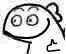### 理论

#### 什么是Q-Learning

Q-learning是一种无模式RL的形式，它也可以被视为异步DP的方法。它通过体验行动的后果，使智能体能够在马尔可夫域中学习以最优方式行动，而无须构建域的映射。智能体在特定状态下尝试行动，并根据其收到的即时奖励或触发以及对其所处状态的值得估计来评估其后果。 通过反复尝试所有状态的所有行动，它可以通过长期折扣奖励来判断总体上最好的行为。

#### 算法

Q : S × A − > R Q:S\times A->R

Q n e w ( s t , a t ) < − ( 1 − α ) Q ( s t , a t ) + α ( r t + γ m a x a Q ( s t + 1 , a ) ) Q^{new}(s_t,a_t)<-(1-\alpha)Q(s_t,a_t)+\alpha(r_t+\gamma max_aQ(s_{t+1},a))

##### 学习率

α \alpha 确定了新获取的信息在多大程度上覆盖旧信息。因子0使得智能体什么都不学习(专门利用先验知识),而因子1使得智能体只考虑最新信息(忽略先验知识，以探索可能性),一般情况下，通常使用恒定的学习率, α t = 0.1 \alpha _t=0.1

#### 例子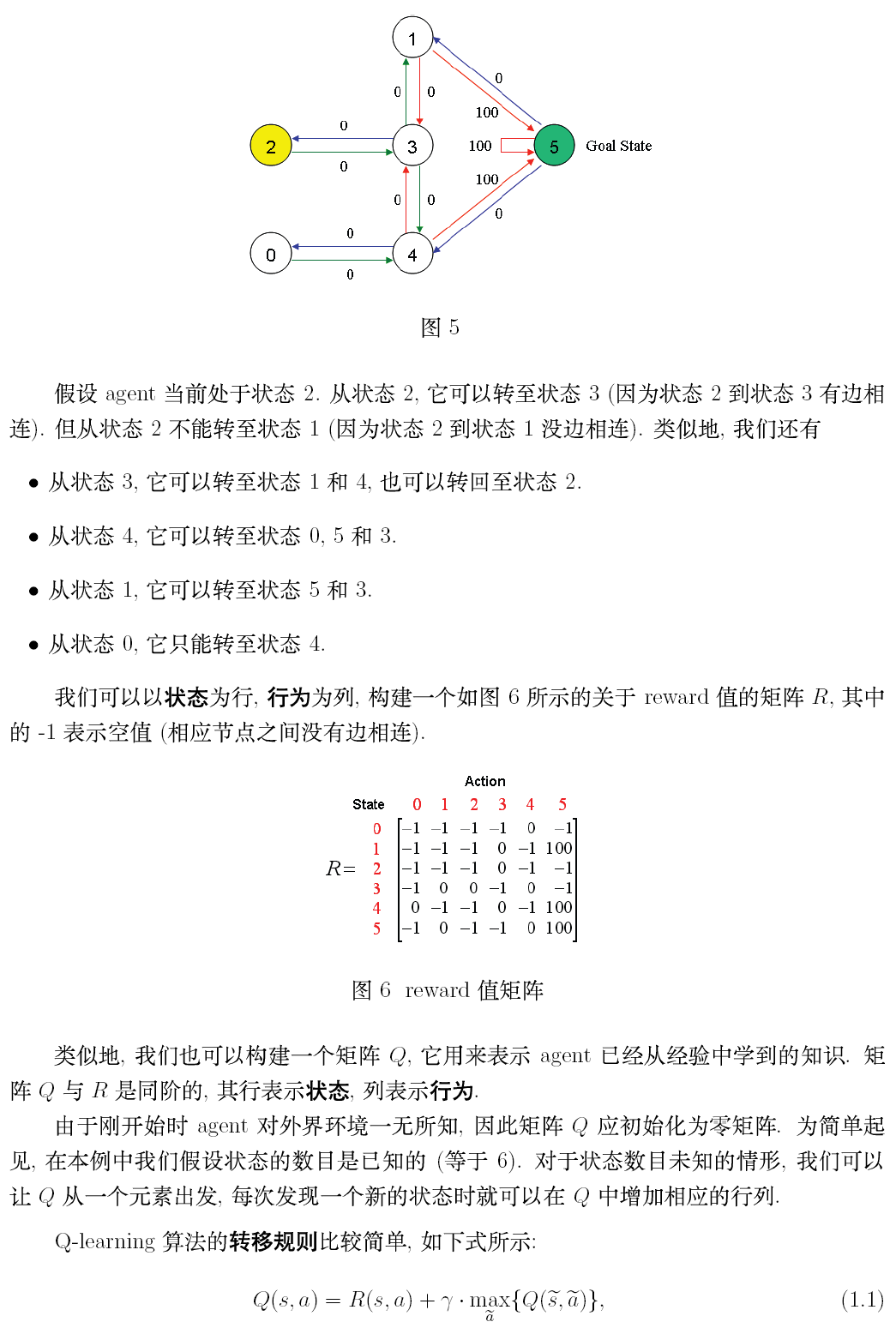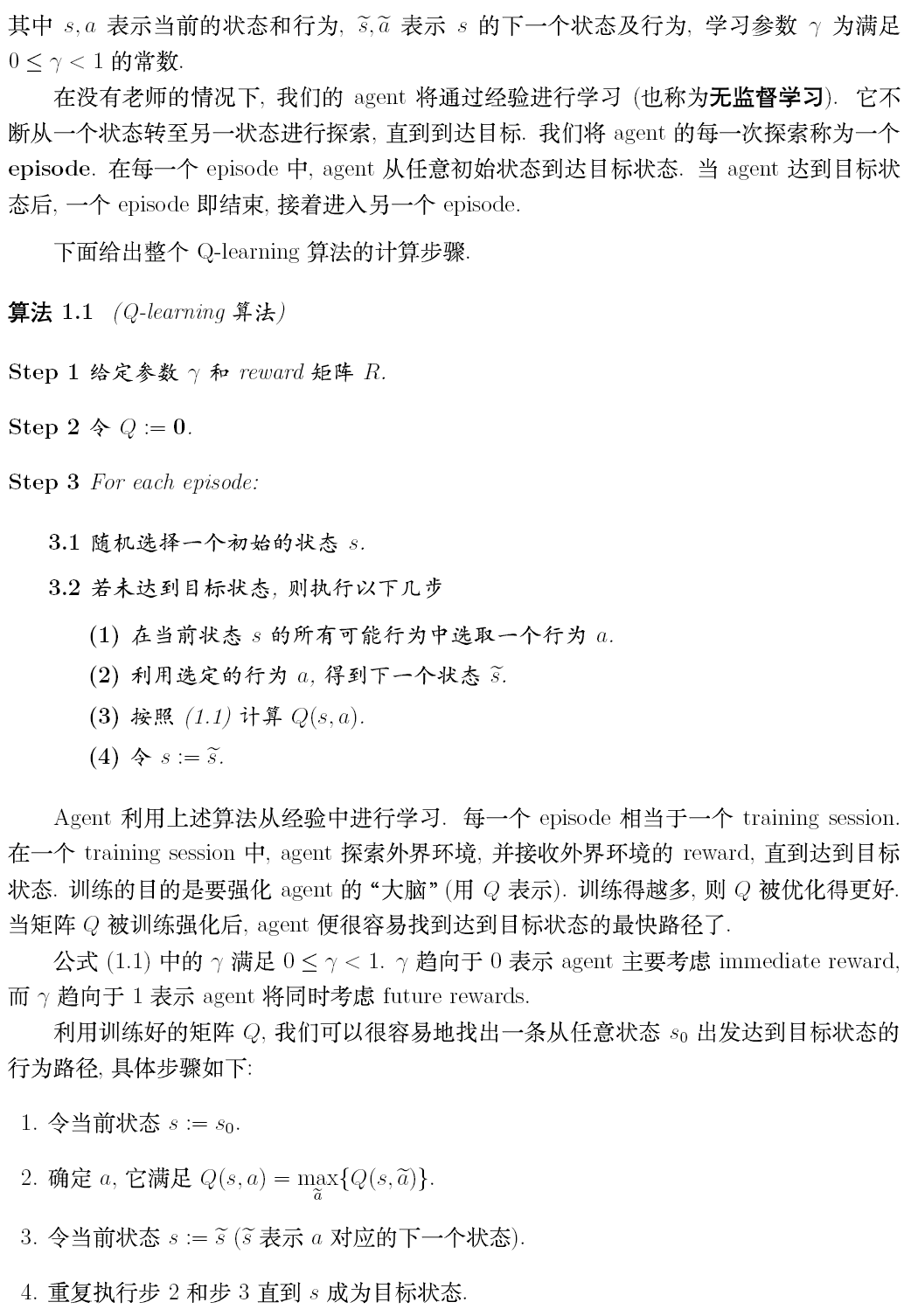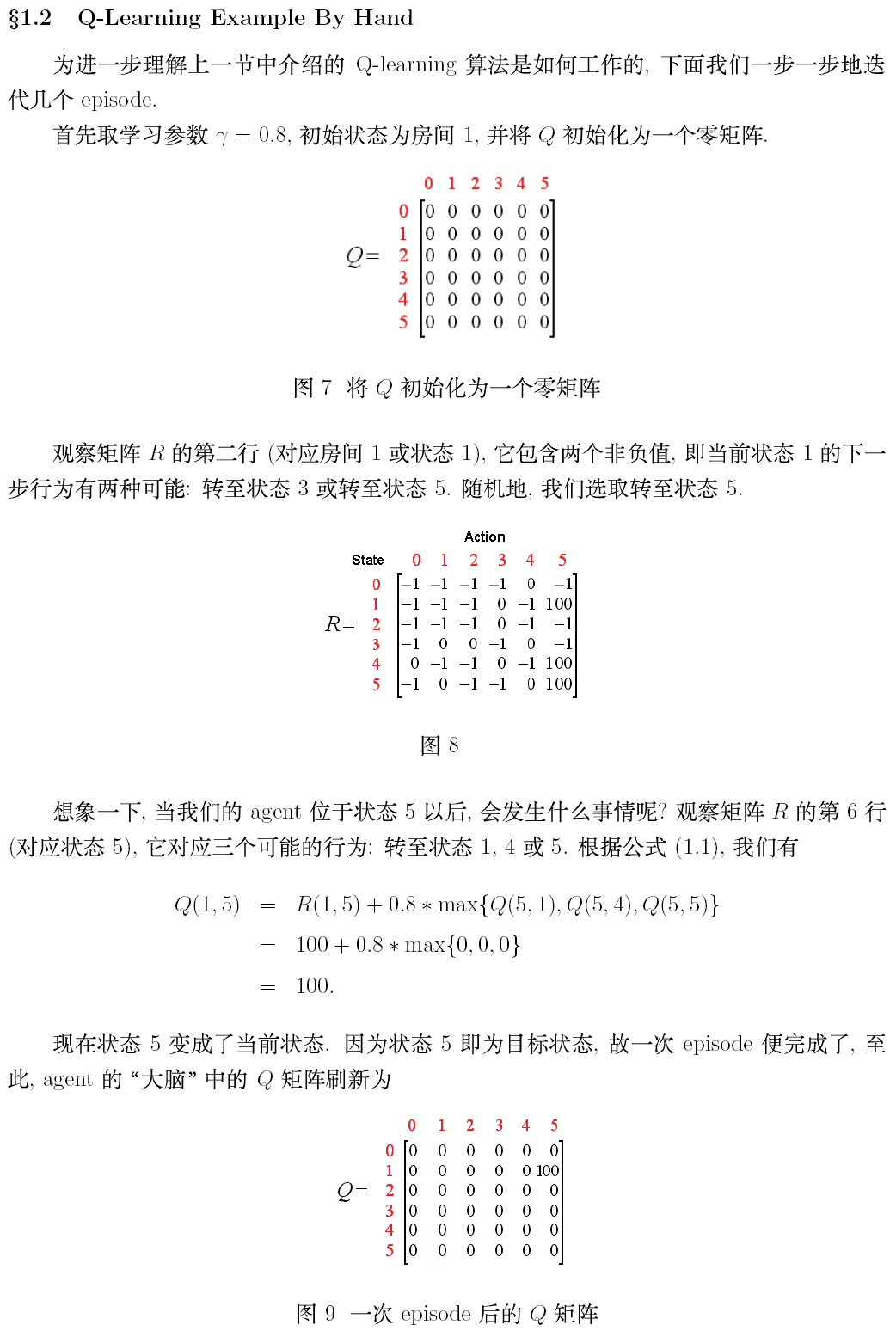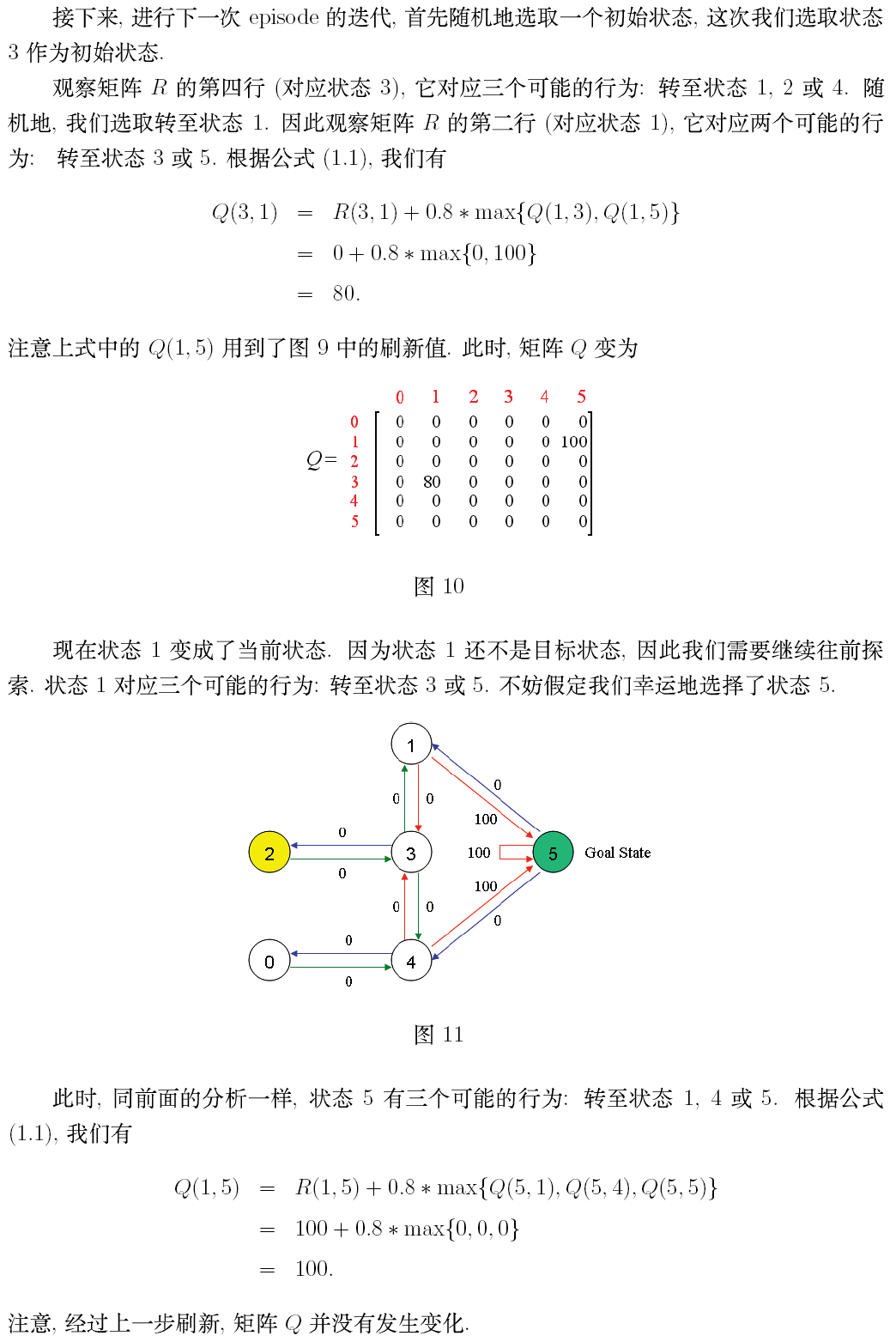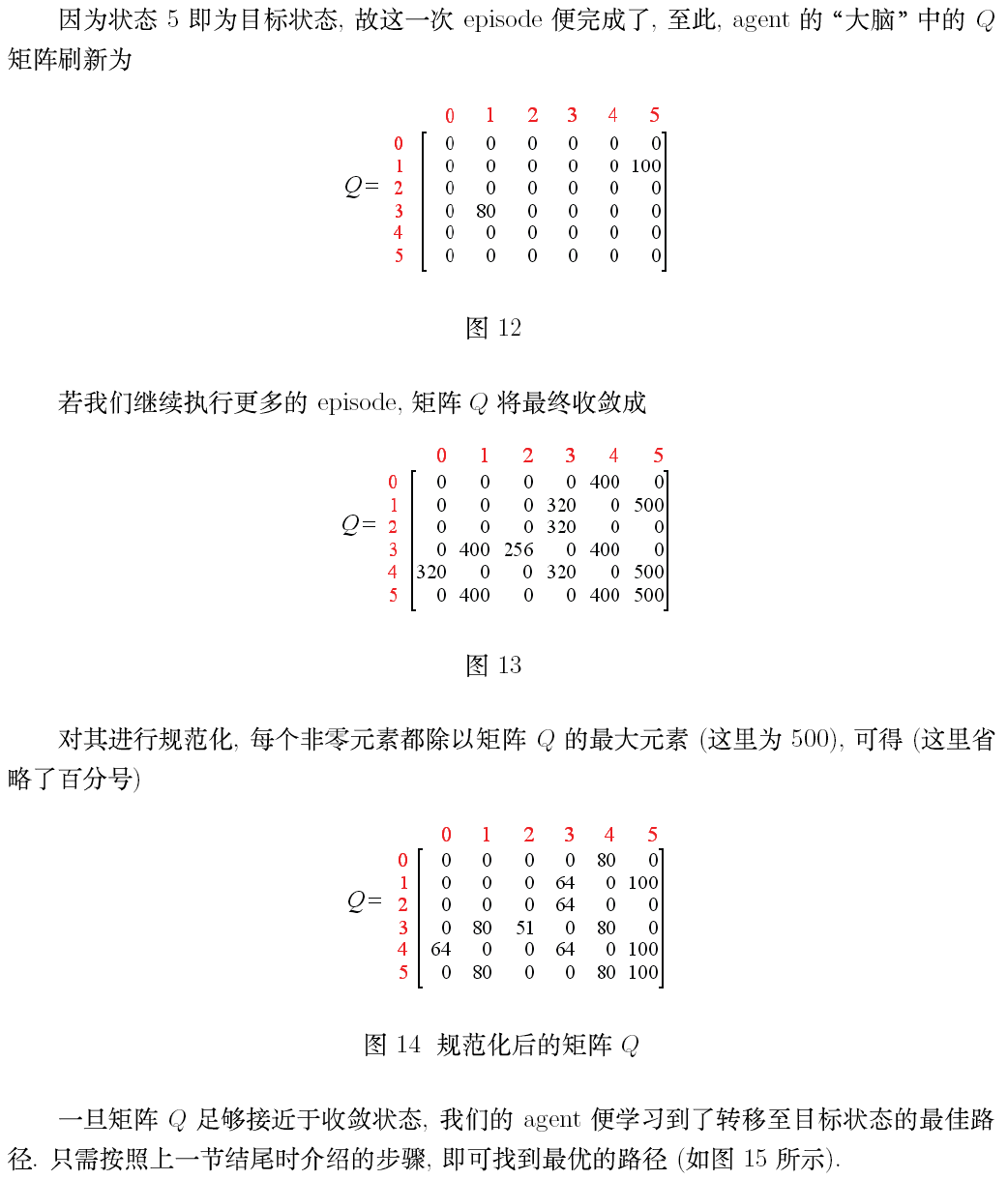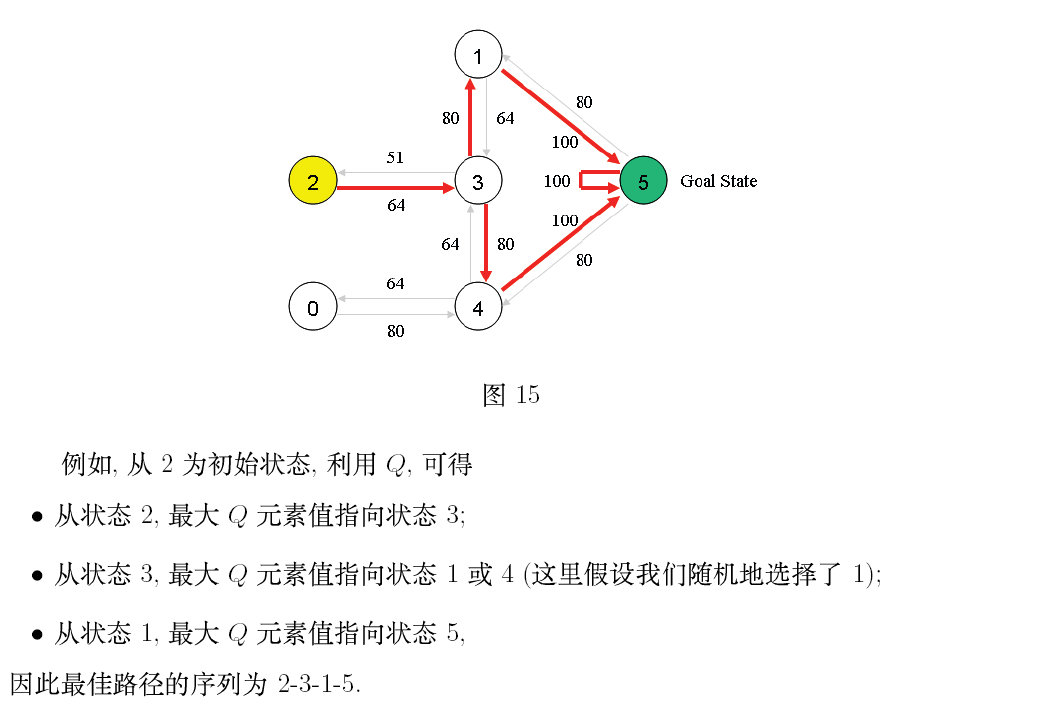### 代码

#### 基础版走迷宫示意图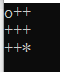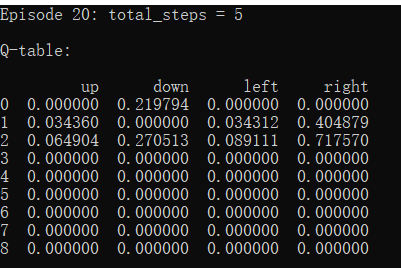#### 升级版走迷宫示意图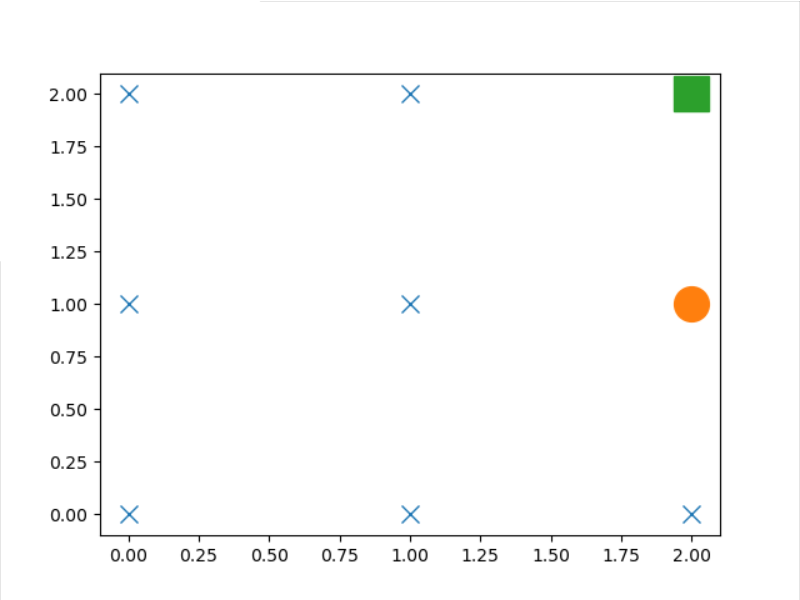#### 完整代码

import numpy as np
import pandas as pd
import time
from matplotlib import pyplot as plt
import os

np.random.seed(3) #固定随机种子，方便调试
N_STATES = 3#迷宫的边长
ACTIONS = ['up','down','left', 'right']#动作,上下左右移动
EPSILON = 0.9#随机率,每10次，选择一次新的随机动作
ALPHA = 0.1#学习率
GAMMA = 0.9#折扣率，当折扣率为0时，则只关注当前奖励，而接近1则关注长期奖励
MAX_EPISODES = 20#最大EPISODERS次数
FRESH_TIME = 0.1#移动时间
X=1#初始坐标x
Y=1#初始坐标Y
target_x=3 #宝藏坐标x
target_y=3 #宝藏坐标y
NOW_STATE=0 #当前位置对应的Q表值

#生成初始Q表
#因为边长为N，每个点有上下左右四个选择，则数量为N*N*4
#N:N
#ACTIONS:动作
def build_q_table(N, actions):
table = pd.DataFrame(
np.zeros((N*N, len(actions))),
columns=actions,
)
#(table)
return table

#选择动作
#EPSILON:选择新动作的概率为1-EPSILON
#state:当前的状态位置(已经转换为一维表上的值)
#q_table:Q表
def choose_action(state, q_table):
state_actions = q_table.iloc[state, :]
if (np.random.uniform() > EPSILON) or ((state_actions == 0).all()):
#当前可以选择的动作表得奖励值都为0(即初始表)时，或者当随机数大于EPSILON时，随机选择一个新动作
action_name = np.random.choice(ACTIONS)
else:
#否则选则当前可选动作表里奖励最大的值
action_name = state_actions.idxmax()
#返回要选择的动作的名字
return action_name

#更新当前位置
def get_env_feedback(x,y,A):
if A == 'up':  #向上移动
#达到上边界，不做变化
if y==1:
y=y
else:
y=y-1
elif A=='down':
#到达下边界，不做变化
if y==N_STATES:
y=y
else:
y=y+1
elif A=='left':
#到达左边界，不做变化
if x==1:
x=x
else:
x=x-1
elif A=='right':
#到达右边界，不做变化
if x==N_STATES:
x=x
else:
x=x+1
R=0
# 判断是否达到终点，到达则将奖励置于1
if (x == target_x) and (y==target_y):
R=1
return x,y,R

# 若到达宝藏位置，则打印本回合的序号和经历的步数。
# 否则打印本次移动后小人的位置（二维世界的当前状态）
def update_env(x,y,target_x, target_y, episode, step_counter):
#做一个以+为点的坐标,*为宝藏
env_list = np.array(['+']*(N_STATES*N_STATES))
env_list=env_list.reshape(N_STATES,N_STATES)
#确定是否达到终点
if (x == target_x) and (y==target_y):
interaction = 'Episode %s: total_steps = %s' % (episode+1, step_counter)
print(interaction+'\n', end='')
time.sleep(2)
step_counter=0
return step_counter
else:
#若未达到终点
#老版本0.01显示图像
env_list[target_x-1][target_y-1]='*'
env_list[x-1][y-1] = 'o'
interaction=''
for a in range(N_STATES):
interaction1 = ''.join(env_list[a,:])
interaction1=interaction1+'\n'
interaction=interaction+interaction1
print(interaction)
time.sleep(0.3)
os.system('cls')
#升级版迷宫图
# label_x=np.array(range(N_STATES))
# label_y=np.array(range(N_STATES))
# label_x = label_x.reshape(N_STATES, 1)
# label_x = np.tile(label_x, (1, N_STATES))
# label_x = label_x.reshape(N_STATES*N_STATES)
# label_y = np.tile(label_y, (N_STATES, 1))
# label_y = label_y.reshape(N_STATES*N_STATES)
# plt.ion()
# plt.cla()
# plt.plot(label_x, label_y, 'x', markersize=10)
# plt.plot(x-1, y-1, 'o', markersize=20)
# plt.plot(target_x-1, target_y-1, 's', markersize=20)
# plt.show()
# plt.pause(0.01)
return step_counter-1

# 强化学习主要的控制器
#step_counter:记录该次episode运行了多少次
#is_terminated:是否是终点
def rl(X_1,Y_1,target_x,target_y):
#c创建初始化零值表
q_table = build_q_table(N_STATES, ACTIONS)
#循环最大次数
step_counter = 0
for episode in range(MAX_EPISODES):
X=X_1
Y=Y_1
is_terminated = False
step_counter=update_env(X,Y,target_x,target_y,episode, step_counter)
#如果不是终点，则进行循环
while not is_terminated:
#当前状态为第x列第y行对应的值
NOW_STATE=int((Y*EPSILON-1)+X-1)
A = choose_action(NOW_STATE, q_table)
#更新当前位置,进行行为并获取奖励和下一次的状态
X_,Y_,R = get_env_feedback(X,Y, A)
q_predict = q_table.loc[NOW_STATE, A]
#确定是否达到终点
if (X_ != target_x) or (Y_!=target_y):
NOW_STATE_ = int((Y_ * EPSILON-1) + X_-1)
q_target = R + GAMMA * q_table.iloc[NOW_STATE_, :].max()
else:
q_target = R
is_terminated = True
#更新Q表
q_table.loc[NOW_STATE, A] += ALPHA * (q_target - q_predict)
#移动到下一个状态
X=X_
Y=Y_
#打印状态
step_counter=update_env(X,Y,target_x,target_y, episode, step_counter+1)
step_counter += 1
return q_table
if __name__ == "__main__":
q_table = rl(X,Y,target_x,target_y)
print('\r\nQ-table:\n')
print(q_table)



### VMware vSphere 8 Update 1 新增功能_sysin.org的博客-程序员秘密

VMware vSphere 8 Update 1 新增功能

### 九度 1482 玛雅人的密码_leobean18的博客-程序员秘密

1482 玛雅人的密码http://ac.jobdu.com/problem.php?pid=1482 http://ac.jobdu.com/oldexamset.php玛雅人有一种密码，如果字符串中出现连续的2012四个数字就能解开密码。给一个长度为N的字符串，（2<= N <=13）该字符串中只含有0,1,2三种数字，问这个字符串要移位几次才能解开密码，每次只能移动相邻的两个数字。例如021

### php-fpm配置文件详解_php-fpm 路径_Asnfy的博客-程序员秘密

php-fpm主配置文件路径：/usr/local/php-fpm/etc/php-fpm.conf#位于安装php安装目录下的etc/目录中，该文件中最后一行将配置文件指向：include=/usr/local/php-fpm/etc/php-fpm.d/*.confphp-fpm子配置文件路径：/usr/local/php-fpm/etc/php-fpm.d/www.conf#php所有...

### 用PHP开发购物车网站（第三篇）：零碎知识(1)_HorseRunningNoStop的博客-程序员秘密

ceil：向上取整数limit：用于在数据库查询时限定选择的记录区间，eg：order by id (asc) limit(4,2);这时数据表是正序排列(id从小到大),limit的意思是(从正序排列的第5条数据开始取2条)order by id desc limit(4,2);这时数据表是倒序排列(id从大到小),limit的意思是(从倒